# Log Analyzer¶

## Overview¶

The log analyzer module reads and parses Varian linear accelerator machine logs, both Dynalogs and Trajectory logs. The module also calculates actual and expected fluences as well as performing gamma evaluations. Data is structured to be easily accessible and easily plottable.

Unlike most other modules of pylinac, the log analyzer module has no end goal. Data is parsed from the logs, but what is done with that info, and which info is analyzed is up to the user.

Features:

• Analyze Dynalogs or Trajectory logs - Either platform is supported. Tlog versions 2.1, 3.0, and 4.0 are supported.
• Read in both .bin and .txt Trajectory log files - Read in the machine data from both .bin and .txt files to get all the information recorded. See the txt attribute.
• Save Trajectory log data to CSV - The Trajectory log binary data format does not allow for easy export of data. Pylinac lets you do that so you can use Excel or other software that you use with Dynalogs.
• Plot or analyze any axis - Every data axis (e.g. gantry, y1, beam holds, MLC leaves) can be accessed and plotted: the actual, expected, and even the difference.
• Calculate fluences and gamma - Besides reading in the MLC positions, pylinac calculates the actual and expected fluence as well as the gamma map; DTA and threshold values are adjustable.
• Anonymize logs - Both dynalogs and trajectory logs can be “anonymized” by removing the Patient ID from the filename(s) and file data.

## Concepts¶

Because the log_analyzer module functions without an end goal, the data has been formatted for easy exploration. However, there are a few concepts that should be grasped before diving in.

• Log Sections - Upon log parsing, all data is placed into data structures. Varian has designated 4 sections for Trajectory logs: Header, Axis Data, Subbeams, and CRC. The Subbeams are only applicable for auto-sequenced beams and all v3.0 logs, and the CRC is specific to the Trajectory log. The Header and Axis Data however, are common to both Trajectory logs and Dynalogs.

Note

Dynalogs do not have explicit sections like the Trajectory logs, but pylinac formats them to have these two data structures for consistency.

• Leaf Indexing & Positions - Varian leaf identification is 1-index based, over against Python’s 0-based indexing. Thus, indexing the first MLC leaf would be , not .

Warning

When slicing or analyzing leaf data, keep the Varian 1-index base in mind.

Leaf data is stored in a dictionary, with the leaf number as the key, from 1 up to the number of MLC leaves. E.g. if the machine has a Millennium 120 standard MLC model, leaf data will have 120 dictionary items from 1 to 120. Leaves from each bank have an offset of half the number of leaves. I.e. leaves A1 and B1 = 1 and 61. Thus, leaves 61-120 correspond to the B-bank, while leaves 1-60 correspond to the A-bank. This can be described by a function $$(A_{n}, B_{n}) = (n, n + N_{leaves}/2)$$, where $$n$$ is the leaf number and $$N_{leaves}$$ is the number of leaves.

• Units - Units follow the Trajectory log specification: linear axes are in cm, rotational axes in degrees, and MU for dose.

Note

Dynalog files are inherently in mm for collimator and gantry axes, tenths of degrees for rotational axes, and MLC positions are not at isoplane. For consistency, Dynalog values are converted to Trajectory log specs, meaning linear axes, both collimator and MLCs are in cm at isoplane, and rotational axes are in degrees. Dynalog MU is always from 0 to 25000 no matter the delivered MU (i.e. it’s relative), unless it was a VMAT delivery, in which case the gantry position is substituted in the dose fraction column.

Warning

Dynalog VMAT files replace the dose fraction column with the gantry position. Unfortunately, because of the variable dose rate of Varian linacs the gantry position is not a perfect surrogate for dose, but there is no other choice. Thus, fluence calculations will use the relative gantry movement as the dose in fluence calculations.

• All data Axes are similar - Log files capture machine data in “control cycles”, aka “snapshots” or “heartbeats”. Let’s assume a log has captured 100 control cycles. Axis data that was captured will all be similar (e.g. gantry, collimator, jaws). They will all have an actual and sometimes an expected value for each cycle. Pylinac formats these as 1D numpy arrays along with a difference array if applicable. Each of these arrays can be quickly plotted for visual analysis. See Axis for more info.

## Running the Demos¶

As usual, the module comes with demo files and methods:

from pylinac import Dynalog
Dynalog.run_demo()


Which will output the following:

Results of file: C:\Users\James\Dropbox\Programming\Python\Projects\pylinac\pylinac\demo_files\AQA.dlg
Average RMS of all leaves: 0.037 cm
Max RMS error of all leaves: 0.076 cm
95th percentile error: 0.088 cm
Number of beam holdoffs: 20
Gamma pass %: 18.65
Gamma average: 0.468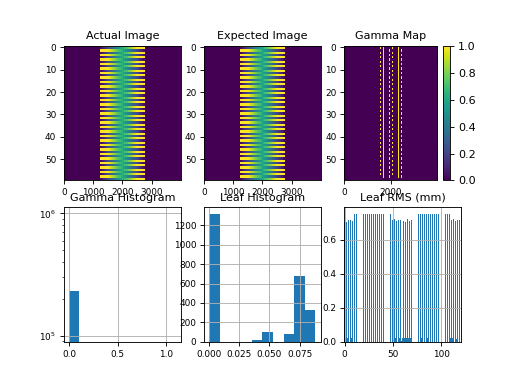Your file location will be different, but the values should be the same. The same can be done using the demo Trajectory log:

from pylinac import TrajectoryLog
TrajectoryLog.run_demo()


Which will give:

Results of file: C:\Users\James\Dropbox\Programming\Python\Projects\pylinac\pylinac\demo_files\Tlog.bin
Average RMS of all leaves: 0.001 cm
Max RMS error of all leaves: 0.002 cm
95th percentile error: 0.002 cm
Number of beam holdoffs: 19
Gamma pass %: 100.00
Gamma average: 0.002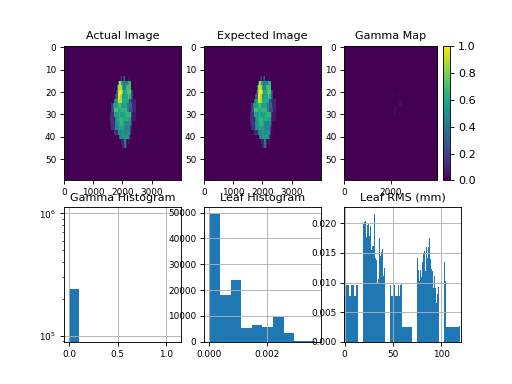Note that you can also save data in a PDF report:

tlog = ...
tlog.publish_pdf('mytlog.pdf')


Logs can be loaded two ways. The first way is through the main helper function load_log().

Note

If you’ve used pylinac versions <1.6 the helper function is new and can be a replacement for MachineLog and MachineLogs, depending on the context as discussed below.

from pylinac import load_log

log_path = "C:/path/to/tlog.bin"


In addition, a folder, ZIP archive, or URL can also be passed:

log2 = load_log('http://myserver.com/logs/2.dlg')


Note

If loading from a URL the object can be a file or ZIP archive.

Pylinac will automatically infer the log type and load it into the appropriate data structures for analysis. The load_log() function is a convenient wrapper around the classes within the log analysis module. However, logs can be instantiated a second way: directly through the classes.

from pylinac import Dynalog, TrajectoryLog

dlog_path = "C:/path/to/dlog.dlg"
dlog = Dynalog(dlog_path)

tlog_path = "C:/path/to/tlog.bin"
tlog = TrajectoryLog(tlog_path)


Loading multiple files is also possible using the load_log() function as listed above. The logs can also be directly instantiated by using MachineLogs. Acceptable inputs include a folder and zip archive.

from pylinac import load_log, MachineLogs

path_to_folder = "C:/path/to/dir"

# from folder; equivalent
logs = MachineLogs(path_to_folder)

# from ZIP archive


## Working with the Data¶

Working with the log data is straightforward once the data structures and Axes are understood (See Concepts for more info). Pylinac follows the data structures specified by Varian for trajectory logs, with a Header and Axis Data structure, and possibly a Subbeams structure if the log is a Trajectory log and was autosequenced. For accessible attributes, see TrajectoryLog. The following sections explore each major section of log data and the data structures pylinac creates to assist in data analysis.

Note

It may be helpful to also read the log specification format in parallel with this guide. It is easier to see that pylinac follows the log specifications and where the info comes from. Log specifications are on MyVarian.com.

Header information is essentially anything that isn’t axis measurement data; it’s metadata about the file, format, machine configuration, etc. Because of the different file formats, there are separate classes for Trajectory log and Dynalog headers. The classes are:

Header attributes are listed in the class API docs by following the above links. For completeness they are also listed here. For Trajectory logs:

• header
• version
• header_size
• sampling_interval
• num_axes
• axis_enum
• samples_per_axis
• num_mlc_leaves
• axis_scale
• num_subbeams
• is_truncated
• num_snapshots
• mlc_model

For Dynalogs the following header information is available:

Example

Let’s explore the header of the demo trajectory log:

>>> tlog = TrajectoryLog.from_demo()
'VOSTL'
2.1
2


### Working with Axis Data¶

Axis data is all the information relating to the measurements of the various machine axes and is accessible under the axis_data attribute. This includes the gantry, collimator, MLCs, etc. Trajectory logs capture more information than Dynalogs, and additionally hold the expected positions not only for MLCs but also for all axes. Every measurement axis has Axis as its base; they all have similar methods to access and plot the data (see Plotting & Saving Axes/Fluences). However, not all attributes are axes. Pylinac adds properties to the axis data structure for ease of use (e.g. the number of snapshots) For Trajectory logs the following attributes are available, based on the TrajectoryLogAxisData class:

• collimator

• gantry

• jaws

Note

The jaws attribute is a data structure to hold all 4 jaw axes; see JawStruct

• couch

Note

The couch attribute is a data structure to hold lateral, longitudinal, etc couch positions; see CouchStruct

• mu

• beam_hold

• control_point

• carriage_A

• carriage_B

• mlc

Note

The mlc attribute is a data structure to hold leaf information; see MLC for attributes and the Working with MLC Data section for more info.

Dynalogs have similar attributes, derived from the DynalogAxisData class:

Example

Let’s access a few axis data attributes:

>>> log = Dynalog.from_demo()
>>> log.axis_data.mu.actual  # a numpy array
array([  0, 100, ...
>>> log.axis_data.num_snapshots
99
>>> log.axis_data.gantry.actual
array([ 180, 180, 180, ...


### Working with MLC Data¶

Although MLC data is acquired and included in Trajectory logs and Dynalogs, it is not always easy to parse. Additionally, a physicist may be interested in the MLC metrics of a log (RMS, etc). Pylinac provides tools for accessing MLC raw data as well as helper methods and properties via the MLC class. Note that this class is consistent between Trajectory logs and Dynalogs. This class is reachable through the axis_data attribute as mlc.

#### Accessing Leaf data¶

Leaf data for any leaf is available under the leaf_axes attribute which is a dict. The leaves are keyed by the leaf number and the value is an Axis. Example:

>>> log = Dynalog.from_demo()
>>> log.axis_data.mlc.leaf_axes.actual  # numpy array of the 'actual' values for leaf #1
array([ 7.56374, ...
>>> log.axis_data.mlc.leaf_axes.difference  # actual values minus the planned values for leaf 84
array([-0.001966, ...


#### MLC helper methods/properties¶

Beyond direct MLC data, pylinac provides a number of helper methods and properties to make working with MLC data easier and more helpful. All the methods are listed in the MLC class, but some examples of use are given here:

>>> log = Dynalog.from_demo()
>>> log.axis_data.mlc.get_error_percentile(percentile=95)  # get an MLC error percentile value
0.08847
>>> log.axis_data.mlc.leaf_moved(12)  # did leaf 12 move during treatment?
False
>>> log.axis_data.mlc.get_RMS_avg()  # get the average RMS error
0.03733
>>> log.axis_data.mlc.get_RMS_avg('A')  # get the average RMS error for bank A
0.03746
>>> log.axis_data.mlc.num_leaves  # the number of MLC leaves
120
>>> log.axis_data.mlc.num_moving_leaves  # the number of leaves that moved during treatment
60


### Working with Fluences¶

Fluences created by the MLCs can also be accessed and viewed. Fluences are accessible under the fluence attribute. There are three subclasses that handle the fluences: The fluence actually delivered is in ActualFluence, the fluence planned is in ExpectedFluence, and the gamma of the fluences is in GammaFluence. Each fluence must be calculated, however pylinac makes reasonable defaults and has a few shortcuts. The actual and expected fluences can be calculated to any resolution in the leaf-moving direction. Some examples:

>>> log = Dynalog.from_demo()
>>> log.fluence.actual.calc_map()  # calculate the actual fluence; returns a numpy array
array([ 0, 0, ...
>>> log.fluence.expected.calc_map(resolution=1)  # calculate at 1mm resolution
array([ 0, 0, ...
>>> log.fluence.gamma.calc_map(distTA=0.5, doseTA=1, resolution=0.1)  # change the gamma criteria
array([ 0, 0, ...
>>> log.fluence.gamma.pass_prcnt  # the gamma passing percentage
99.82
>>> log.fluence.gamma.avg_gamma  # the average gamma value
0.0208


### Plotting & Saving Axes/Fluences¶

Each and every axis of the log can be accessed as a numpy array and/or plotted. For each axis the “actual” array/plot is always available. Dynalogs only have expected values for the MLCs. Trajectory logs have the actual and expected values for all axes. Additionally, if an axis has actual and expected arrays, then the difference is also available.

Example of plotting the MU actual:

import pylinac

log = pylinac.TrajectoryLog.from_demo()
log.axis_data.mu.plot_actual()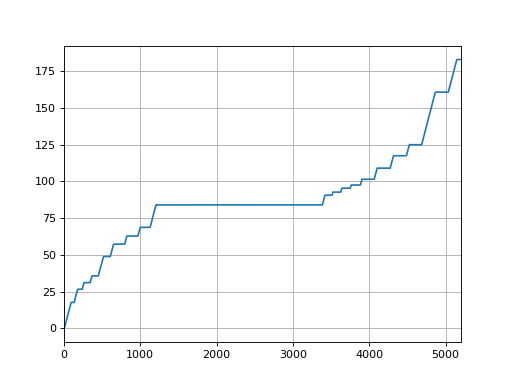Plot the Gantry difference:

log.axis_data.gantry.plot_difference()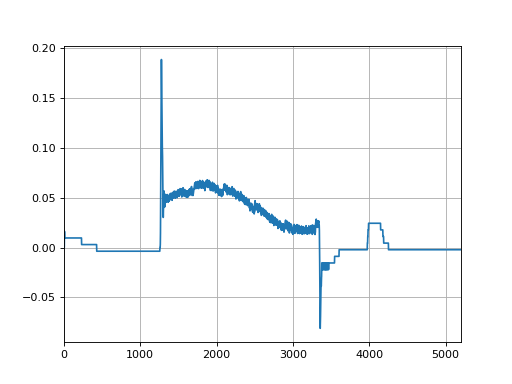Axis plots are just as easily saved:

log.axis_data.gantry.save_plot_difference(filename='gantry diff.png')


Now, lets plot the actual fluence:

log.fluence.actual.calc_map()
log.fluence.actual.plot_map()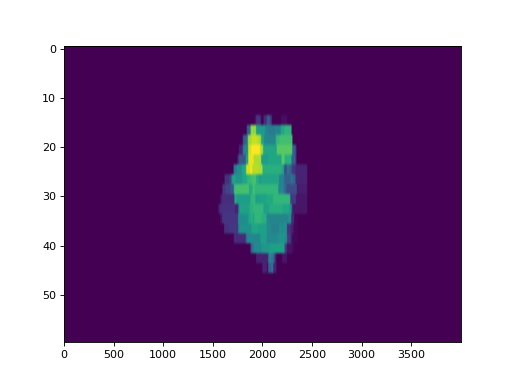And the fluence gamma. But note we must calculate the gamma first, passing in any DoseTA or DistTA parameters:

log.fluence.gamma.calc_map()
log.fluence.gamma.plot_map()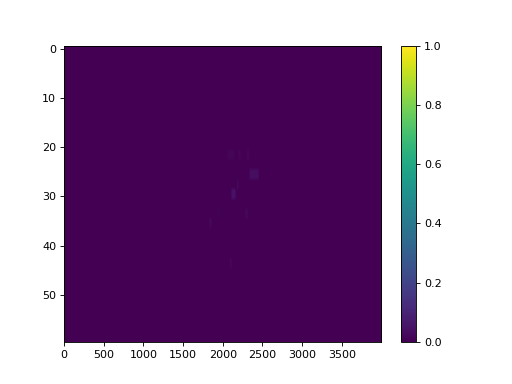Additionally, you can calculate and view the fluences of subbeams if you’re working with trajectory logs:

log.subbeams.fluence.gamma.calc_map()
log.subbeams.fluence.actual.plot_map()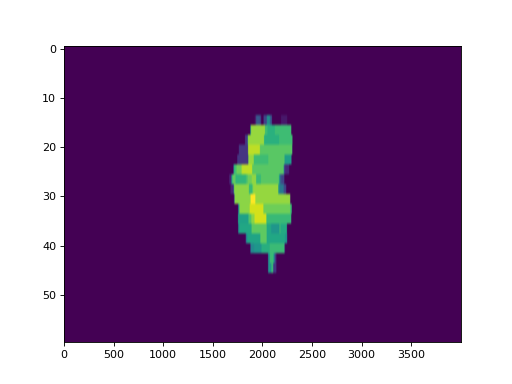## Converting Trajectory logs to CSV¶

If you already have the log files, you obviously have a record of treatment. However, trajectory logs are in binary format and are not easily readable without tools like pylinac. You can save trajectory logs in a more readable format through the to_csv() method. This will write the log to a comma-separated variable (CSV) file, which can be read with Excel and many other programs. You can do further or specialized analysis with the CSV files if you wish, without having to use pylinac:

log = TrajectoryLog.from_demo()
log.to_csv()


## Anonymizing Logs¶

Machine logs can be anonymized two ways. The first is using the anonymize() method, available to both Trajectory logs and Dynalogs. Example script:

tlog = TrajectoryLog.from_demo()
tlog.anonymize()
dlog = Dynalog.from_demo()
dlog.anonymize()


The other way is the use the module function anonymize(). This function will anonymize a single log file or a whole directory. If you plan on anonymizing a lot of logs, use this method as it is threaded and is much faster:

from pylinac.log_analyzer import anonymize

log_file = 'path/to/tlog.bin'
anonymize(log_file)
log_dir = 'path/to/log/folder'
anonymize(log_dir) # VERY fast


## Batch Processing¶

Batch processing/loading of log files is helpful when dealing with one file at a time is too cumbersome. Pylinac allows you to load logs of an entire directory via MachineLogs; individual log files can be accessed, and a handful of batch methods are included.

Example

Let’s assume all of your logs for the past week are in a folder. You’d like to quickly see what the average gamma is of the files:

>>> from pylinac import MachineLogs
>>> log_dir = r"C:\path\to\log\directory"
>>> logs = MachineLogs(log_dir)
>>> logs.avg_gamma(resolution=0.2)
0.03  # or whatever


You can also append to MachineLogs to have two or more different folders combined:

>>> other_log_dir = r"C:\different\path"
>>> logs.append(other_log_dir)


Trajectory logs in a MachineLogs instance can also be converted to CSV, just as for a single instance of TrajectoryLog:

>>> logs.to_csv()  # only converts trajectory logs; dynalogs are already basically CSV files


Note

Batch processing methods (like avg_gamma() can take a while if numerous logs have been loaded, so be patient. You can also use the verbose=True argument in batch methods to see how the process is going.

## API Documentation¶

### Main classes¶

These are the classes a typical user may interface with.

pylinac.log_analyzer.load_log(file_or_dir: str, exclude_beam_off: bool = True, recursive: bool = True) → Union[pylinac.log_analyzer.TrajectoryLog, pylinac.log_analyzer.Dynalog, pylinac.log_analyzer.MachineLogs][source]

Load a log file or directory of logs, either dynalogs or Trajectory logs.

Parameters: file_or_dir (str) – String pointing to a single log file or a directory that contains log files. exclude_beam_off (bool) – Whether to include snapshots where the beam was off. recursive (bool) – Whether to recursively search a directory. Irrelevant for single log files.
pylinac.log_analyzer.anonymize(source: str, inplace: bool = False, destination: bool = None, recursive: bool = True)[source]

Quickly anonymize an individual log or directory of logs. For directories, threaded execution is performed, making this much faster (10-20x) than loading a MachineLogs instance of the folder and using the .anonymize() method.

Note

Because MachineLog instances are not overly memory-efficient, you may run into MemoryError issues. To avoid this, try not to anonymize more than ~3000 logs at once.

Parameters: source (str) – Points to a local log file (e.g. .dlg or .bin file) or to a directory containing log files. inplace (bool) – Whether to edit the file itself, or created an anonymized copy and leave the original. destination (str, None) – Where the put the anonymized logs. Must point to an existing directory. If None, will place the logs in their original location. recursive (bool) – Whether to recursively enter sub-directories below the root source folder.
class pylinac.log_analyzer.Dynalog(filename, exclude_beam_off: bool = True)[source]

Bases: pylinac.log_analyzer.LogBase

header
axis_data
fluence
a_logfile

Path of the A* dynalog file.

b_logfile

Path of the B* dynalog file.

num_beamholds

Return the number of times the beam was held.

classmethod from_demo(exclude_beam_off: bool = True)[source]

Load and instantiate from the demo dynalog file included with the package.

static run_demo()[source]

Run the Dynalog demo.

publish_pdf(filename: str, notes: str = None, metadata: dict = None, open_file: bool = False)[source]

Publish (print) a PDF containing the analysis and quantitative results.

Parameters: filename ((str, file-like object}) – The file to write the results to. notes (str, list of strings) – Text; if str, prints single line. If list of strings, each list item is printed on its own line. open_file (bool) – Whether to open the file using the default program after creation. metadata (dict) – Extra data to be passed and shown in the PDF. The key and value will be shown with a colon. E.g. passing {‘Author’: ‘James’, ‘Unit’: ‘TrueBeam’} would result in text in the PDF like: ————– Author: James Unit: TrueBeam ————–
static identify_other_file(first_dlg_file: str, raise_find_error: bool = True) → str[source]

Return the filename of the corresponding dynalog file.

For example, if the A*.dlg file was passed in, return the corresponding B*.dlg filename. Can find both A- and B-files.

Parameters: first_dlg_file (str) – The absolute file path of the dynalog file. raise_find_error (bool) – Whether to raise an error if the file isn’t found. The absolute file path to the corresponding dynalog file. str
class pylinac.log_analyzer.TrajectoryLog(filename: Union[str, BinaryIO], exclude_beam_off: bool = True)[source]

Bases: pylinac.log_analyzer.LogBase

A class for loading and analyzing the data of a Trajectory log.

header
Type: ~pylinac.log_analyzer.TrajectoryLogHeader, which has the following attributes:
axis_data
Type: ~pylinac.log_analyzer.TrajectoryLogAxisData
fluence
Type: ~pylinac.log_analyzer.FluenceStruct
subbeams
Type: ~pylinac.log_analyzer.SubbeamManager
txt_filename

The name of the associated .txt file for the .bin file. The file may or may not be available.

classmethod from_demo(exclude_beam_off: bool = True)[source]

Load and instantiate from the demo trajetory log file included with the package.

static run_demo()[source]

Run the Trajectory log demo.

to_csv(filename: Optional[str] = None) → str[source]

Write the log to a CSV file.

Parameters: filename (None, str) – If None (default), the CSV filename will be the same as the filename of the log. If a string, the filename will be named so. The full filename of the newly created CSV file. str
publish_pdf(filename: Union[str, BinaryIO], metadata: dict = None, notes: Union[str, list] = None, open_file: bool = False)[source]

Publish (print) a PDF containing the analysis and quantitative results.

Parameters: filename ((str, file-like object}) – The file to write the results to. notes (str, list of strings) – Text; if str, prints single line. If list of strings, each list item is printed on its own line. open_file (bool) – Whether to open the file using the default program after creation. metadata (dict) – Extra data to be passed and shown in the PDF. The key and value will be shown with a colon. E.g. passing {‘Author’: ‘James’, ‘Unit’: ‘TrueBeam’} would result in text in the PDF like: ————– Author: James Unit: TrueBeam ————–
num_beamholds

Return the number of times the beam was held.

is_hdmlc

Whether the machine has an HDMLC or not.

class pylinac.log_analyzer.MachineLogs(folder: str, recursive: bool = True)[source]

Bases: list

Read in machine logs from a directory. Inherits from list. Batch methods are also provided.

Parameters: folder (str) – The directory of interest. Will walk through and process any logs, Trajectory or dynalog, it finds. Non-log files will be skipped. recursive (bool) – Whether to walk through subfolders of passed directory. Only used if folder is a valid log directory.

Examples

>>> log_folder = r'C:\path\log\directory'
>>> logs = MachineLogs(log_folder)


Batch methods include determining the average gamma and average gamma pass value:

>>> logs.avg_gamma()
>>> 0.05 # or whatever it is
>>> logs.avg_gamma_pct()
>>> 97.2

classmethod from_zip(zfile: str)[source]

Instantiate from a ZIP archive.

Parameters: zfile (str) – Path to the zip archive.
num_logs

The number of logs currently loaded.

num_tlogs

The number of Trajectory logs currently loaded.

num_dlogs

The number of Trajectory logs currently loaded.

load_folder(directory: str, recursive: bool = True)[source]

Load log files from a directory and append to existing list.

Parameters: directory (str, None) – The directory of interest. If a string, will walk through and process any logs, Trajectory or dynalog, it finds. Non-log files will be skipped. If None, files must be loaded later using .load_dir() or .append(). recursive (bool) – If True (default), will walk through subfolders of passed directory. If False, will only search root directory.
report_basic_parameters() → None[source]

Report basic parameters of the logs.

• Number of logs
• Average gamma value of all logs
• Average gamma pass percent of all logs
append(obj, recursive: bool = True) → None[source]

Append a log. Overloads list method.

Parameters: obj (str, Dynalog, TrajectoryLog) – If a string, must point to a log file. If a directory, must contain log files. If a Dynalog or Trajectory log instance, then simply appends. recursive (bool) – Whether to walk through subfolders of passed directory. Only applicable if obj was a directory.
avg_gamma(doseTA: Union[int, float] = 1, distTA: Union[int, float] = 1, threshold: Union[int, float] = 0.1, resolution: Union[int, float] = 0.1) → float[source]

Calculate and return the average gamma of all logs. See calc_map() for further parameter info.

avg_gamma_pct(doseTA: Union[int, float] = 1, distTA: Union[int, float] = 1, threshold: Union[int, float] = 0.1, resolution: Union[int, float] = 0.1) → float[source]

Calculate and return the average gamma pass percent of all logs. See calc_map() for further parameter info.

to_csv() → List[str][source]

Write trajectory logs to CSV. If there are both dynalogs and trajectory logs, only the trajectory logs will be written. File names will be the same as the original log file names.

Returns: A list of all the filenames of the newly created CSV files. list
anonymize(inplace: bool = False, suffix: Optional[str] = None)[source]

Save anonymized versions of the logs.

For dynalogs, this replaces the patient ID in the filename(s) and the second line of the log with ‘Anonymous<suffix>. This will rename both A* and B* logs if both are present in the same directory.

For trajectory logs, the patient ID in the filename is replaced with Anonymous<suffix> for the .bin file. If the associated .txt file is in the same directory it will similarly replace the patient ID in the filename with Anonymous<suffix>. Additionally, the Patient ID row will be replaced with Patient ID: Anonymous<suffix>.

Note

Anonymization is only available for logs loaded locally (i.e. not from a URL or a data stream). To anonymize such a log it must be first downloaded or written to a file, then loaded in.

Note

Anonymization is done to the log file itself. The current instance(s) of MachineLog will not be anonymized.

Parameters: inplace (bool) – If False (default), creates an anonymized copy of the log(s). If True, renames and replaces the content of the log file. suffix (str, optional) – An optional suffix that is added after Anonymous to give specificity to the log. A list containing the paths to the newly written files. list
class pylinac.log_analyzer.Graph[source]

Bases: enum.Enum

An enumeration.

GAMMA = 'gamma'
HISTOGRAM = 'histogram'
RMS = 'rms'
class pylinac.log_analyzer.MLCBank[source]

Bases: enum.Enum

An enumeration.

A = 'A'
B = 'B'
BOTH = 'both'
class pylinac.log_analyzer.Fluence[source]

Bases: enum.Enum

An enumeration.

ACTUAL = 'actual'
EXPECTED = 'expected'
GAMMA = 'gamma'
class pylinac.log_analyzer.TreatmentType[source]

Bases: enum.Enum

An enumeration.

STATIC_IMRT = 'Static IMRT'
DYNAMIC_IMRT = 'Dynamic IMRT'
VMAT = 'VMAT'
IMAGING = 'Imaging'

### Supporting Classes¶

You generally won’t have to interface with these unless you’re doing advanced behavior.

class pylinac.log_analyzer.Metadata(stream: BinaryIO, num_axes: int)[source]

Bases: object

Metadata field for Trajectory logs v4.0+.

Warning

The TrueBeam log file spec says that there is a reserved section of the same size as v3.0 following this section. That is NOT TRUE. It is actually offset by the size of the metadata; meaning 1024 - (64 + num_axes * 8) - 745.

class pylinac.log_analyzer.Axis(actual: numpy.ndarray, expected: Optional[numpy.ndarray] = None)[source]

Bases: object

Represents an ‘Axis’ of a Trajectory log or dynalog file, holding actual and potentially expected and difference values.

Parameters are Attributes
Parameters: actual (numpy.ndarray) – The array of actual position values. expected (numpy.ndarray, optional) – The array of expected position values. Not applicable for dynalog axes other than MLCs.
difference

Return an array of the difference between actual and expected positions.

Returns: Array the same length as actual/expected. numpy.ndarray
plot_actual() → None[source]

Plot the actual positions as a matplotlib figure.

plot_expected() → None[source]

Plot the expected positions as a matplotlib figure.

plot_difference() → None[source]

Plot the difference of positions as a matplotlib figure.

class pylinac.log_analyzer.MLC(log_type, snapshot_idx: Optional[numpy.ndarray] = None, jaw_struct=None, hdmlc: bool = False, subbeams=None)[source]

Bases: object

The MLC class holds MLC information and retrieves relevant data about the MLCs and positions.

Parameters: log_type (Dynalog, TrajectoryLog) – snapshot_idx (array, list) – The snapshots to be considered for RMS and error calculations (can be all snapshots or just when beam was on). jaw_struct (Jaw_Struct) – hdmlc (boolean) – If False (default), indicates a regular MLC model (e.g. Millennium 120). If True, indicates an HD MLC model (e.g. Millennium 120 HD).
leaf_axes

The dictionary is keyed by the leaf number, with the Axis as the value.

Warning

Leaf numbers are 1-index based to correspond with Varian convention.

Type: dict containing Axis
classmethod from_dlog(dlog, jaws, snapshot_data: numpy.ndarray, snapshot_idx: Union[list, numpy.ndarray])[source]

Construct an MLC structure from a Dynalog

classmethod from_tlog(tlog, subbeams, jaws, snapshot_data, snapshot_idx, column_iter)[source]

Construct an MLC instance from a Trajectory log.

num_pairs

Return the number of MLC pairs.

num_leaves

Return the number of MLC leaves.

num_snapshots

Return the number of snapshots used for MLC RMS & Fluence calculations.

Warning

This number may not be the same as the number of recorded snapshots in the log since the snapshots where the beam was off may not be included. See MachineLog.load()

num_moving_leaves

Return the number of leaves that moved.

moving_leaves

Return an array of the leaves that moved during treatment.

add_leaf_axis(leaf_axis: pylinac.log_analyzer.LeafAxis, leaf_num: int) → None[source]

Add a leaf axis to the MLC data structure.

Parameters: leaf_axis (LeafAxis) – The leaf axis to be added. leaf_num (int) – The leaf number. Warning Leaf numbers are 1-index based to correspond with Varian convention.
leaf_moved(leaf_num: int) → bool[source]

Return whether the given leaf moved during treatment.

Parameters: leaf_num (int) –

Warning

Leaf numbers are 1-index based to correspond with Varian convention.

pair_moved(pair_num: int) → bool[source]

Return whether the given pair moved during treatment.

If either leaf moved, the pair counts as moving.

Parameters: pair_num (int) –

Warning

Pair numbers are 1-index based to correspond with Varian convention.

get_RMS_avg(bank: pylinac.log_analyzer.MLCBank = <MLCBank.BOTH: 'both'>, only_moving_leaves: bool = False)[source]

Return the overall average RMS of given leaves.

Parameters: bank – Specifies which bank(s) is desired. only_moving_leaves (boolean) – If False (default), include all the leaves. If True, will remove the leaves that were static during treatment. Warning The RMS and error will nearly always be lower if all leaves are included since non-moving leaves have an error of 0 and will drive down the average values. Convention would include all leaves, but prudence would use only the moving leaves to get a more accurate assessment of error/RMS. float
get_RMS_max(bank: pylinac.log_analyzer.MLCBank = <MLCBank.BOTH: 'both'>) → float[source]

Return the overall maximum RMS of given leaves.

Parameters: bank – Specifies which bank(s) is desired. float
get_RMS_percentile(percentile: Union[int, float] = 95, bank: pylinac.log_analyzer.MLCBank = <MLCBank.BOTH: 'both'>, only_moving_leaves: bool = False)[source]

Return the n-th percentile value of RMS for the given leaves.

Parameters: percentile (int) – RMS percentile desired. bank – Specifies which bank(s) is desired. only_moving_leaves (boolean) – If False (default), include all the leaves. If True, will remove the leaves that were static during treatment. Warning The RMS and error will nearly always be lower if all leaves are included since non-moving leaves have an error of 0 and will drive down the average values. Convention would include all leaves, but prudence would use only the moving leaves to get a more accurate assessment of error/RMS.
get_RMS(leaves_or_bank: Union[str, pylinac.log_analyzer.MLCBank, Iterable[T_co]]) → numpy.ndarray[source]

Return an array of leaf RMSs for the given leaves or MLC bank.

Parameters: leaves_or_bank (sequence of numbers, {'a', 'b', 'both'}) – If a sequence, must be a sequence of leaf numbers desired. If a string, it specifies which bank (or both) is desired. An array for the given leaves containing the RMS error. numpy.ndarray
get_leaves(bank: pylinac.log_analyzer.MLCBank = <MLCBank.BOTH: 'both'>, only_moving_leaves: bool = False) → list[source]

Return a list of leaves that match the given conditions.

Parameters: bank ({'A', 'B', 'both'}) – Specifies which bank(s) is desired. only_moving_leaves (boolean) – If False (default), include all the leaves. If True, will remove the leaves that were static during treatment.
get_error_percentile(percentile: Union[int, float] = 95, bank: pylinac.log_analyzer.MLCBank = <MLCBank.BOTH: 'both'>, only_moving_leaves: bool = False) → float[source]

Calculate the n-th percentile error of the leaf error.

Parameters: percentile (int) – RMS percentile desired. bank ({'A', 'B', 'both'}) – Specifies which bank(s) is desired. only_moving_leaves (boolean) – If False (default), include all the leaves. If True, will remove the leaves that were static during treatment. Warning The RMS and error will nearly always be lower if all leaves are included since non-moving leaves have an error of 0 and will drive down the average values. Convention would include all leaves, but prudence would use only the moving leaves to get a more accurate assessment of error/RMS.
create_error_array(leaves: Sequence[int], absolute: bool = True) → numpy.ndarray[source]

Create and return an error array of only the leaves specified.

Parameters: leaves (sequence) – Leaves desired. absolute (bool) – If True, (default) absolute error will be returned. If False, error signs will be retained. An array of size leaves-x-num_snapshots numpy.ndarray
create_RMS_array(leaves: Sequence[int]) → numpy.ndarray[source]

Create an RMS array of only the leaves specified.

Parameters: leaves (sequence) – Leaves desired. An array of size leaves-x-num_snapshots numpy.ndarray
leaf_under_y_jaw(leaf_num: int) → bool[source]

Return a boolean specifying if the given leaf is under one of the y jaws.

Parameters: leaf_num (int) –
get_snapshot_values(bank_or_leaf: Union[pylinac.log_analyzer.MLCBank, Iterable[T_co]] = <MLCBank.BOTH: 'both'>, dtype: str = 'actual') → numpy.ndarray[source]

Retrieve the snapshot data of the given MLC bank or leaf/leaves

Parameters: bank_or_leaf (str, array, list) – If a str, specifies what bank (‘A’, ‘B’, ‘both’). If an array/list, specifies what leaves (e.g. [1,2,3]) dtype ({'actual', 'expected'}) – The type of MLC snapshot data to return. An array of shape (number of leaves - x - number of snapshots). E.g. for an MLC bank and 500 snapshots, the array would be (60, 500). ndarray
plot_mlc_error_hist(show: bool = True) → None[source]

Plot an MLC error histogram.

save_mlc_error_hist(filename: str, **kwargs) → None[source]

Save the MLC error histogram to file.

plot_rms_by_leaf(show: bool = True) → None[source]

Plot RMSs by leaf.

save_rms_by_leaf(filename: str, **kwargs) → None[source]

Save the RMS-leaf to file.

class pylinac.log_analyzer.DynalogHeader(dlogdata)[source]
version

The Dynalog version letter.

Type: str
patient_name

Patient information.

Type: str
plan_filename

Filename if using standalone. If using Treat =<6.5 will produce PlanUID, Beam Number. Not yet implemented for this yet.

Type: str
tolerance

Plan tolerance.

Type: int
num_mlc_leaves

Number of MLC leaves.

Type: int
clinac_scale

Clinac scale; 0 -> Varian scale, 1 -> IEC 60601-2-1 scale

Type: int
class pylinac.log_analyzer.DynalogAxisData(log, dlogdata)[source]

Bases: object

num_snapshots

Number of snapshots recorded.

Type: int
mu

Current dose fraction

Note

This can be gantry rotation under certain conditions. See Dynalog file specs.

Type: Axis
previous_segment_num

Previous segment number, starting with zero.

Type: Axis
beam_hold

Beam hold state; 0 -> holdoff not asserted (beam on), 1 -> holdoff asserted, 2 -> carriage in transition

Type: Axis
beam_on

Beam on state; 1 -> beam is on, 0 -> beam is off

Type: Axis
prior_dose_index

Previous segment dose index or previous segment gantry angle.

Type: Axis
next_dose_index

Next segment dose index.

Type: Axis
gantry

Gantry data in degrees.

Type: Axis
collimator

Collimator data in degrees.

Type: Axis
jaws

Jaw data structure. Data in cm.

Type: Jaw_Struct
carriage_A

Carriage A data. Data in cm.

Type: Axis
carriage_B

Carriage B data. Data in cm.

Type: Axis
mlc

MLC data structure. Data in cm.

Type: MLC

class pylinac.log_analyzer.TrajectoryLogHeader(file: BinaryIO)[source]

Bases: object

version : str
Log version.
sampling_interval : int
Sampling interval in milliseconds.
num_axes : int
Number of axes sampled.
axis_enum : int
samples_per_axis : numpy.ndarray
Number of samples per axis; 1 for most axes, for MLC it’s # of leaves and carriages.
num_mlc_leaves : int
Number of MLC leaves.
axis_scale : int
Axis scale; 1 -> Machine scale, 2 -> Modified IEC 61217.
num_subbeams : int
Number of subbeams, if autosequenced.
is_truncated : int
Whether log was truncated due to space limitations; 0 -> not truncated, 1 -> truncated
num_snapshots : int
Number of snapshots, cycles, heartbeats, or whatever you’d prefer to call them.
mlc_model : int
The MLC model; 2 -> NDS 120 (e.g. Millennium), 3 -> NDS 120 HD (e.g. Millennium 120 HD)
class pylinac.log_analyzer.TrajectoryLogAxisData(log, file, subbeams)[source]

Bases: object

collimator : Axis
Collimator data in degrees.
gantry : Axis
Gantry data in degrees.
jaws : Jaw_Struct
Jaw data structure. Data in cm.
couch : Couch_Struct
Couch data structure. Data in cm.
mu : Axis
MU data in MU.
beam_hold : Axis
Beam hold state. Beam pauses (e.g. Beam Off button pressed) are not recorded in the log. Data is automatic hold state. 0 -> Normal; beam on. 1 -> Freeze; beam on, dose servo is temporarily turned off. 2 -> Hold; servo holding beam. 3 -> Disabled; beam on, dose servo is disable via Service.
control_point : Axis
Current control point.
carriage_A : Axis
Carriage A data in cm.
carriage_B : Axis
Carriage B data in cm.
mlc : MLC
MLC data structure; data in cm.
class pylinac.log_analyzer.SubbeamManager(file, header)[source]

Bases: object

One of 4 subsections of a trajectory log. Holds a list of Subbeams; only applicable for auto-sequenced beams.

post_hoc_metadata(axis_data)[source]

From the Axis Data, perform post-hoc analysis and set metadata to the subbeams. Gives the subbeams more information, as not much is given directly in the logs.

class pylinac.log_analyzer.Subbeam(file, log_version: float)[source]

Bases: object

Data structure for trajectory log “subbeams”. Only applicable for auto-sequenced beams.

control_point

Internally-defined marker that defines where the plan is currently executing.

Type: int
mu_delivered

Dose delivered in units of MU.

Type: float
rad_time

Type: float
sequence_num

Sequence number of the subbeam.

Type: int
beam_name

Name of the subbeam.

Type: str
gantry_angle

Median gantry angle of the subbeam.

collimator_angle

Median collimator angle of the subbeam.

jaw_x1

Median X1 position of the subbeam.

jaw_x2

Median X2 position of the subbeam.

jaw_y1

Median Y1 position of the subbeam.

jaw_y2

Median Y2 position of the subbeam.

class pylinac.log_analyzer.FluenceStruct(mlc_struct=None, mu_axis: pylinac.log_analyzer.Axis = None, jaw_struct=None)[source]

Bases: object

Structure for data and methods having to do with fluences.

actual

The actual fluence delivered.

expected

The expected, or planned, fluence.

gamma

The gamma structure regarding the actual and expected fluences.

class pylinac.log_analyzer.FluenceBase(mlc_struct=None, mu_axis: pylinac.log_analyzer.Axis = None, jaw_struct=None)[source]

Bases: object

An abstract base class to be used for the actual and expected fluences.

array

An array representing the fluence map; will be num_mlc_pairs-x-400/resolution. E.g., assuming a Millennium 120 MLC model and a fluence resolution of 0.1mm, the resulting matrix will be 60-x-4000.

Type: numpy.ndarray
resolution

The resolution of the fluence calculation; -1 means calculation has not been done yet.

Type: int, float
Parameters: mlc_struct (MLC_Struct) – mu_axis (BeamAxis) – jaw_struct (Jaw_Struct) –
is_map_calced(raise_error: bool = False) → bool[source]

Return a boolean specifying whether the fluence has been calculated.

calc_map(resolution: float = 0.1, equal_aspect: bool = False) → numpy.ndarray[source]

Calculate a fluence pixel map.

Image calculation is done by adding fluence snapshot by snapshot, and leaf pair by leaf pair. Each leaf pair is analyzed separately. First, to optimize, it checks if the leaf is under the y-jaw. If so, the fluence is left at zero; if not, the leaf (or jaw) ends are determined and the MU fraction of that snapshot is added to the total fluence. All snapshots are iterated over for each leaf pair until the total fluence matrix is built.

Parameters:
• resolution (int, float) – The resolution in mm of the fluence calculation in the leaf-moving direction.
• equal_aspect (bool) –

If True, make the y-direction the same resolution as x. If False, the y-axis will be equal to the number of leaves.

returns: A numpy array reconstructing the actual fluence of the log. The size will be the number of MLC pairs by 400 / resolution since the MLCs can move anywhere within the 40cm-wide linac head opening. numpy.ndarray
plot_map(show: bool = True) → None[source]

Plot the fluence; the fluence (pixel map) must have been calculated first.

save_map(filename: str, **kwargs) → None[source]

Save the fluence map figure to a file.

class pylinac.log_analyzer.ActualFluence(mlc_struct=None, mu_axis: pylinac.log_analyzer.Axis = None, jaw_struct=None)[source]

The actual fluence object

Parameters: mlc_struct (MLC_Struct) – mu_axis (BeamAxis) – jaw_struct (Jaw_Struct) –
class pylinac.log_analyzer.ExpectedFluence(mlc_struct=None, mu_axis: pylinac.log_analyzer.Axis = None, jaw_struct=None)[source]

The expected fluence object.

Parameters: mlc_struct (MLC_Struct) – mu_axis (BeamAxis) – jaw_struct (Jaw_Struct) –
class pylinac.log_analyzer.GammaFluence(actual_fluence: pylinac.log_analyzer.ActualFluence, expected_fluence: pylinac.log_analyzer.ExpectedFluence, mlc_struct)[source]

Gamma object, including pixel maps of gamma, binary pass/fail pixel map, and others.

array

The gamma map. Only available after calling calc_map()

Type: numpy.ndarray
passfail_array

The gamma pass/fail map; pixels that pass (<1.0) are set to 0, while failing pixels (>=1.0) are set to 1.

Type: numpy.ndarray
distTA

The distance to agreement value used in gamma calculation.

Type: int, float
doseTA

The dose to agreement value used in gamma calculation.

Type: int, float
threshold

The threshold percent dose value, below which gamma was not evaluated.

Type: int, float
pass_prcnt

The percent of pixels passing gamma (<1.0).

Type: float
avg_gamma

The average gamma value.

Type: float
Parameters: actual_fluence (ActualFluence) – The actual fluence object. expected_fluence (ExpectedFluence) – The expected fluence object. mlc_struct (MLC_Struct) – The MLC structure, so fluence can be calculated from leaf positions.
calc_map(doseTA: Union[int, float] = 1, distTA: Union[int, float] = 1, threshold: Union[int, float] = 0.1, resolution: Union[int, float] = 0.1, calc_individual_maps: bool = False) → numpy.ndarray[source]

Calculate the gamma from the actual and expected fluences.

The gamma calculation is based on Bakai et al eq.6, which is a quicker alternative to the standard Low gamma equation.

Parameters: doseTA (int, float) – Dose-to-agreement in percent; e.g. 2 is 2%. distTA (int, float) – Distance-to-agreement in mm. threshold (int, float) – The dose threshold percentage of the maximum dose, below which is not analyzed. resolution (int, float) – The resolution in mm of the resulting gamma map in the leaf-movement direction. calc_individual_maps (bool) – Not yet implemented. If True, separate pixel maps for the distance-to-agreement and dose-to-agreement are created. A num_mlc_leaves-x-400/resolution numpy array. numpy.ndarray
plot_map(show: bool = True)[source]

Plot the fluence; the fluence (pixel map) must have been calculated first.

histogram(bins: Optional[list] = None) → Tuple[numpy.ndarray, numpy.ndarray][source]

Return a histogram array and bin edge array of the gamma map values.

Parameters: bins (sequence) – The bin edges for the gamma histogram; see numpy.histogram for more info. histogram (numpy.ndarray) – A 1D histogram of the gamma values. bin_edges (numpy.ndarray) – A 1D array of the bin edges. If left as None, the class default will be used (self.bins).
plot_histogram(scale: str = 'log', bins: Optional[list] = None, show: bool = True) → None[source]

Plot a histogram of the gamma map values.

Parameters: scale ({'log', 'linear'}) – Scale of the plot y-axis. bins (sequence) – The bin edges for the gamma histogram; see numpy.histogram for more info.
save_histogram(filename: str, scale: str = 'log', bins: Optional[list] = None, **kwargs) → None[source]

Save the histogram plot to file.

plot_passfail_map() → None[source]

Plot the binary gamma map, only showing whether pixels passed or failed.

class pylinac.log_analyzer.JawStruct(x1: pylinac.log_analyzer.HeadAxis, y1: pylinac.log_analyzer.HeadAxis, x2: pylinac.log_analyzer.HeadAxis, y2: pylinac.log_analyzer.HeadAxis)[source]

Bases: object

Jaw Axes data structure.

x1
Type: Axis
y1
Type: Axis
x2
Type: Axis
y2
Type: Axis
class pylinac.log_analyzer.CouchStruct(vertical: pylinac.log_analyzer.CouchAxis, longitudinal: pylinac.log_analyzer.CouchAxis, lateral: pylinac.log_analyzer.CouchAxis, rotational: pylinac.log_analyzer.CouchAxis, pitch: Optional[pylinac.log_analyzer.CouchAxis] = None, roll: Optional[pylinac.log_analyzer.CouchAxis] = None)[source]

Bases: object

Couch Axes data structure.

vert
Type: Axis
long
Type: Axis
latl
Type: Axis
rotn
Type: Axis
class pylinac.log_analyzer.NotALogError[source]

Bases: OSError

Machine log error. Indicates that the passed file is not a valid machine log file.

class pylinac.log_analyzer.NotADynalogError[source]

Bases: OSError

Dynalog error. Indicates that the passed file is not a valid dynalog file.

class pylinac.log_analyzer.DynalogMatchError[source]

Bases: OSError`

Dynalog error. Indicates that the associated file of the dynalog passed in (A file if B passed in & vic versa) cannot be found. Ensure associated file is in the same folder and has the same name as the passed file, except the first letter.